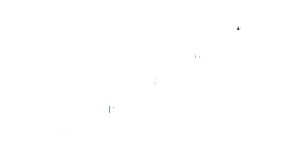Home > Math Shortcuts > Brain Trigger #22 – Sample Letters puzzles question

# Brain Trigger #22 – Sample Letters puzzles question

Now here we will discuss few question answers on Letters puzzles which are very common in competitive exams. Questions like Letters puzzles will help you to prepare for your competitive exams. To solve these questions you need to apply your maths skills. You can also apply your learning skills of Short-cut Tricks.

We try to bring together all types of short cut methods on Letters puzzles for every topic here in this website. what you need to do is to apply these tricks in Letters puzzles problem to solve. However it’s possible that you may not need any tricks to solve few questions.

We will discuss here a question on Letters puzzles. Read the provided question on Letters puzzles very carefully and try your best to solve the question. Answer of this question will be provided below. If you solve the problem then check your answer with us. If your answer doesn’t match with our answer then check below for the solution.

Each page contains a question on Letters puzzles and also its solution with explanation. If you want to check other questions then click on Next/Previous link below.

Let’s starts the question and answer session. Today’s question is:

What should be the missing letter replaces in the question mark ?Scroll down below to know the answer of this question. You can take as much time as you want to answer the problem. But try to solve this question as quickly as you can.

(Scroll down to get the answer)

(Scroll down to get the answer)

(Scroll down to get the answer)

(Scroll down to get the answer)

Now answer of the above question will be discuss here. If you already try the problem and got an solution then check your answer with our solution. Well Done if you correctly answer the previous question, but don’t worry if you are not able to answer the question correctly. Here is the answer of the question with explanation.

You can solve in this way…….

A   B  C   D  E  F G H  I  J   K  L   M N  O P   Q  R  S   T   U  V   W  X  Y   Z
1    2   3   4   5  6 7  8   9 10 11 12 13 14 15 16 17 18 19 20 21 22 23 24 25 26

1 X 1 = 1 = A
2 X 2 = 4 = D
3 X 3 = 9 = I
4 X 4 = 16 = P
5 X 5 = 25 = Y

Y replaces in the question mark

 << PREVIOUS QUESTION NEXT QUESTION >>

We provide few other questions on other topics also. Click on the Next/Previous link to get more Question Answer session.